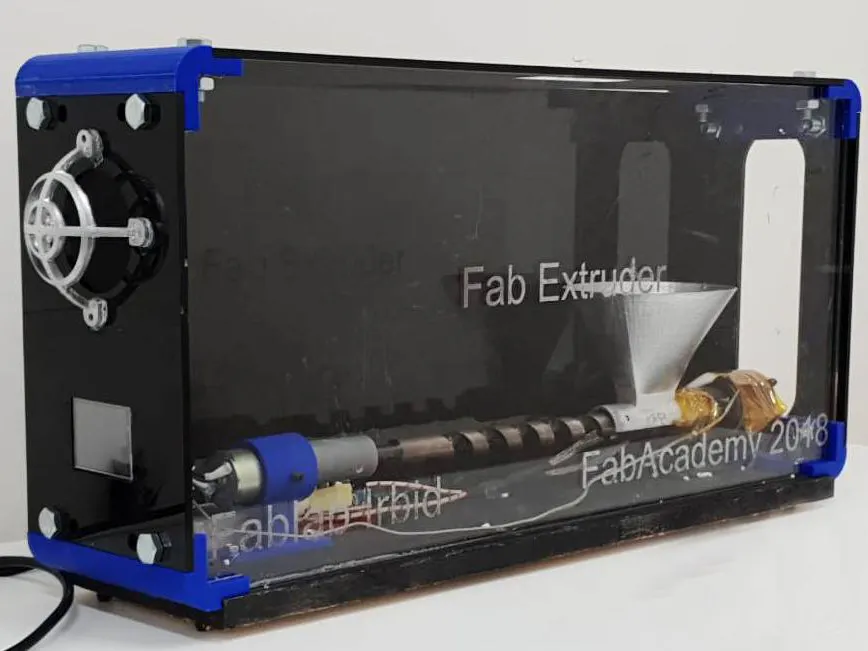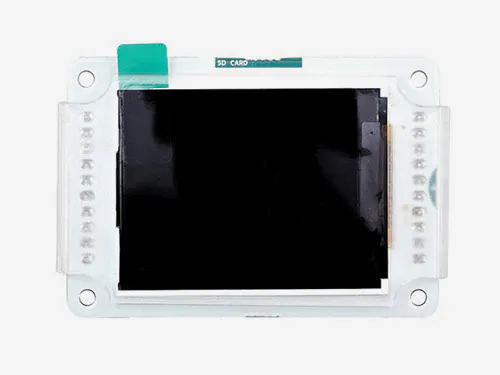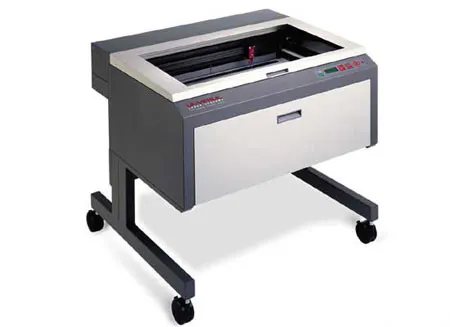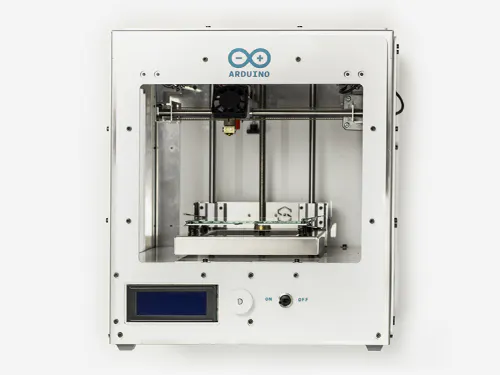# Filament Recycling Extruder "Fab Extruder"

This project introduces a functional extruder at a reasonable price, which can be affordable to the fablab community and others.## Things used in this project

### Hardware components

 DC motor (generic)
×1
 K-type thermocouple
×1Arduino LCD Screen
×1
 Solid State Relay
×1

### Software apps and online servicesArduino IDEAutodesk Fusion 360

### Hand tools and fabrication machinesLaser cutter (generic)Arduino Materia 101

## Code

### Filament Recycling Extruder

Arduino
```#include "Nextion.h"
#include <max6675.h>

'[[[p
"

NexNumber n0 = NexNumber(0, 8, "n0");

NexSlider h0 = NexSlider(0, 3, "h0");
NexSlider h1 = NexSlider(0, 4, "h1");

int thermo_so_pin  = 12;
int thermo_cs_pin  = 3;
int thermo_sck_pin = 13;
int temp=20;

int heatprev;
int heater=5;
int motor=6;
MAX6675 thermocouple(thermo_sck_pin, thermo_cs_pin, thermo_so_pin);

uint32_t heat = 20;
uint32_t Speed = 0;

NexTouch *nex_listen_list[] =

{

&h0,
h1,

NULL

};&

void setup()
{
pinMode(heater, OUTPUT);
pinMode(motor,OUTPUT);
nexInit();
}
void loop()
{

h0.getValue(&heat);
h1.getValue(&Speed);
delay(100);

if(heat==heatprev){
n0.setValue(temp);
heatprev=heat;
}
else {
n0.setValue(heat);
heatprev=heat;
}

nexLoop(nex_listen_list);
delay(100);

if(temp<heat){
digitalWrite(heater,HIGH);
}
else if(temp >heat+5){
digitalWrite(heater,LOW);
}

if (temp>heat){
analogWrite(motor,Speed);
}

else if(temp<heat-5) {
analogWrite(motor,0);
}

}
```

## Credits

Thanks to Qusai Malahmeh .